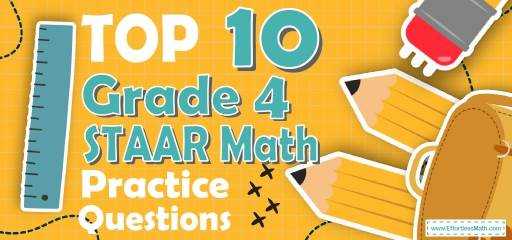# Top 10 4th Grade STAAR Math Practice Questions## 4th Grade STAAR Math Practice Questions

1- Joe has 855 crayons. What is this number rounded to the nearest ten? _________

2- Peter’s pencil is $$\frac{12}{100}$$ of a meter long. What is the length, in meters, of Peter’s pencil written as a decimal?

A. 0.12

B. 1.02

C. 1.2

D. 12.100

3- There are 7 days in a week. There are 28 days in the month of February. How many times as many days are there in February than are in one week?

A. 4

B. 7

C. 21

D. 35

4- A football team is buying new uniforms. Each uniform costs $24. The team wants to buy 14 uniforms. Which equation represents a way to find the total cost of the uniforms? A. $$(20 × 10) + (4 × 4) = 200 + 16$$ B. $$(20 × 4) + (10 × 4) = 80 + 40$$ C. $$(24 × 10) + (24 × 4) = 240 + 96$$ D. $$(24 × 4) + (4 × 14) = 96 + 56$$ 5- A number sentence such as $$31 + Z = 98$$ can be called an equation. If this equation is true, then which of the following equations is not true? A. $$98 – 31 = Z$$ B. $$98 – Z = 31$$ C. $$Z – 31 = 98$$ D. $$Z + 31 = 98$$ 6- Circle a reasonable measurement for the angle: A. $$35^\circ$$ B. $$90^\circ$$ C. $$180^\circ$$ D. $$240^\circ$$ 7- Ella described a number using these clues: Three-digit odd numbers that have a 6 in the hundreds place and a 3 in the tens place Which number could fit Ella’s description? A. 627 B. 637 C. 632 D. 636 8- Tam has 390 cards. He wants to put them in boxes of 30 cards. How many boxes does he need? A. 7 B. 9 C. 11 D. 13 9- If this clock shows a time in the morning, what time was it 6 hours and 30 minutes ago? A. 07:45 AM B. 05:45 AM C. 07:45 PM D. 05:45 PM 10- Use the table below to answer the question. The students in the fourth-grade class voted for their favorite sport. Which bar graph shows the results of the student’s votes? A. B. C. D. ## Best 4th Grade STAAR Math Exercise Resource for 2022 ## Answers: 1- 860 We round the number up to the nearest ten if the last digit in the number is 5, 6, 7, 8, or 9. We round the number down to the nearest ten if the last digit in the number is 1, 2, 3, or 4. If the last digit is 0, then we do not have to do any rounding, because it is already to the ten. Therefore, a rounded number of 855 to the nearest ten is 860. 2- A $$\frac{12}{100}$$ is equal to 0.12 3- A 7 days = 1 week 28 days $$= (28 ÷ 7)= 4$$ weeks 4- C Football team should buy 14 uniforms that each uniform cost$24 so they should pay (14 $$×$$ $24)$336.
Therefore, choice C is correct answer:
$$(24 × 10) + (24 × 4) =24(10+14) =24×14 = 336$$

5- C
These: $$98 – 31 = Z$$
$$98 – Z = 31$$
And $$Z + 31 = 98$$ are equal.

6- A
This angle is less than $$90^\circ$$. just choice A shows an angle less than $$90^\circ$$.

7- B
Three-digit odd numbers that have a 6 in the hundreds place and a 3 in the tens place are 631, 633, 635, 637, 639. 637 is one of the alternatives.

8- D
Tam wants to divide his 390 cards into boxes of 30 cards. So he needs $$390÷30=13$$ boxes.

9- C
Subtract hours: $$2 – 6 = -4$$
Subtract the minutes: $$15 – 30 = – 15$$
The minutes are less than 0, so:
• Add 60 to minutes ( $$-15 +60 =45$$ minutes)
• Subtract 1 from hours $$(-4 – 1 = -5)$$ the hours are less than 0, add 24: $$(24 – 5 =19)$$
The answer is 19:45 that is equal to 7:45

10- A

## The Best Books to Ace the 4th Grade STAAR Math Test

### What people say about "Top 10 4th Grade STAAR Math Practice Questions - Effortless Math: We Help Students Learn to LOVE Mathematics"?

No one replied yet.

X
30% OFF

Limited time only!

Save Over 30%

SAVE $5 It was$16.99 now it is \$11.99